# IIT JAM Chemistry - MCQ Test 4

## 60 Questions MCQ Test MOCK Test Series for IIT JAM Chemistry | IIT JAM Chemistry - MCQ Test 4

Description
This mock test of IIT JAM Chemistry - MCQ Test 4 for Chemistry helps you for every Chemistry entrance exam. This contains 60 Multiple Choice Questions for Chemistry IIT JAM Chemistry - MCQ Test 4 (mcq) to study with solutions a complete question bank. The solved questions answers in this IIT JAM Chemistry - MCQ Test 4 quiz give you a good mix of easy questions and tough questions. Chemistry students definitely take this IIT JAM Chemistry - MCQ Test 4 exercise for a better result in the exam. You can find other IIT JAM Chemistry - MCQ Test 4 extra questions, long questions & short questions for Chemistry on EduRev as well by searching above.
QUESTION: 1

Solution:
QUESTION: 2

Solution:
QUESTION: 3

### The relationship been Osmotic pressure at 273K when 10g Glucose (P1), 10g Urea (P2), 10g Sucrose (P3) are dissolved in 250 Ml of water

Solution:
QUESTION: 4

Solution A contains 7g/L of MgCl2 and Solution B contains 7g/L of NaCl. At room temp, Osmotic pressure of

Solution:
QUESTION: 5

When a substance is dissolved in solvent, its vapor pressure is decreased. It brings

Solution:

When a solute dissolves in water, the vapour pressure of solvent decreases and because of this boiling point increases and freezing point decreases.

QUESTION: 6

At a given temperature, total vapor pressure in Torr of a mixture of volatile components A and B is given by

p = 120 – 75XB

Hence, vapor pressure of pure A and B respectively (in Torr) are:

Solution:
QUESTION: 7

The equilibrium constant is 6.0 × 10–4 for the N2O2→ 2NO reaction. If the concentration of nitrogen is 0.10 mol/L and concentration of oxygen is 0.20 mol/L at equilibrium. Then the concentration of nitric oxide at equilibrium is:

Solution:
QUESTION: 8

Some solid NH4HS is placed in a flask containing 0.5 atm of NH3, what would be pressure of NH3 and H2S when equilibrium is reached:

NH4HS(g)→ NH3(g) + H2S(g),  KP = 0.11

Solution:
QUESTION: 9

Ratio of sigma and pi bonds is maximum in

Solution:
QUESTION: 10

For the following three reactions (I), (II) and (III) equilibrium constants are given

(I) CO(g) + H2O(g)→ CO2(g) + H2(g); K1
(II) CH4(g) + H2O(g)→ CO(g) + 3H2(g); K2
(III) CH4(g) + 2H2O(g)→ CO2(g) + 4H2(g); K3

Which of the following relations is correct:

Solution:
QUESTION: 11

XY2 dissociates as XY2→ XY(g) + Y(g) when the initial pressure of XY2 is 600 mmHg, the total equilibrium pressure is 800mm Hg. Calculate K for the reaction assuming that the volume of the system remain unchanged:

Solution:
QUESTION: 12

A 50 mL sample of 0.01M Ba(OH)2 is titrated with 0.01 M HCl. The solution at equivalent point is

Solution:
QUESTION: 13

Which of the following fluorides is angular:

Solution:
QUESTION: 14

Based on the following information,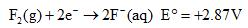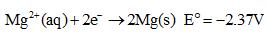Which of the following chemical species is the strongest reducing agent?

Solution:
QUESTION: 15

Which molecule has zero bond order:

Solution:
QUESTION: 16

Which of the following processes involves increasing in bond order:

Solution:
QUESTION: 17

At room temperature, HCl is a gas while HF is a liquid because:

Solution:
QUESTION: 18

The bond order and bond type in the C2 molecule are, respectively:

Solution:
QUESTION: 19

The major product formed in the following reacting is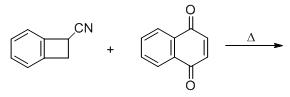Solution:
QUESTION: 20

Predict the condition A and the structure of the major product B in the following sequence.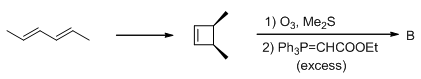Solution:
QUESTION: 21

The major product B is: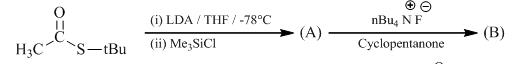Solution:
QUESTION: 22

The major product P is: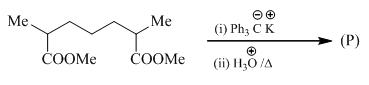Solution:
QUESTION: 23

The conditions A-B, required for the following pericyclic are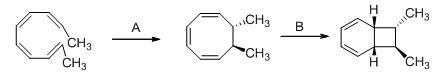Solution:
QUESTION: 24

The major product P is: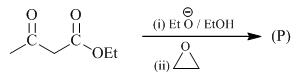Solution:
QUESTION: 25

Solubility of BaSO4 in aqueous solution is 1 × 10–5 M. Hence, solubility in 0.1 M BaCl2 is:

Solution:

BaSO₄ ---------> Ba²⁺ + SO₄²⁻
According to ICE table
BaSO₄ -----------------> Ba²⁺ + SO₄²⁻
I                     0.1 M    0
C                    +s       +s
E                    s + 0.1    s
therefore we can write
ksp = ( s + 0.01) . s
as the value of ksp is very small as compared to silver ions initial concentration
we can use approximation
s + 0.1 ≈ 0.1
Ksp = 0.01 . s
s = 1 X 10⁻⁵ / 0.1
s = 0.0001 mol/ L
s = 1 X 10⁻4 mol/ L

QUESTION: 26

pH of a mixture of 1 M benzoic acid (pKa = 4.20) and 1 M sodium benzoate is 4.5. In 300 mL buffer, benzoic acid is:

Solution:
QUESTION: 27

58.5 g of NaCl and 180g of glucose were separately dissolved in 1L of water. Identify the correct statements regarding the elevation in boiling point of solution.

Solution:
QUESTION: 28

Elevation in boiling point of solution of 13.44g og CuCl2 in 1 Kg of water will be (M.wt = 134.4, Kb =0.52Kmolal-1, assume it is a strong electrolyte)

Solution:
QUESTION: 29

At 700 K, the equilibrium constant Kp, for the reaction 2 SO3 (g) ⇌ 2 SO2 (g) + O2 Is 1.8 X 10-3 kPa. What is the numerical value in moles per liter of Kc for the reaction at the same temperature?

Solution:
QUESTION: 30

0.1 mol of N2O4(g) was sealed in a tube under 1 atmospheric pressure at 25oC. Calculate the no of mole of NO2(g) present if equilibrium is reached after sometime (Kp = 0.14)

Solution:
*Multiple options can be correct
QUESTION: 31

The compound pair which shows correct order of angle:

(I) NH3> PH3,    (II) H2S < H2O,           (III) NO2+ >NO2    (IV)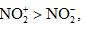Solution:
*Multiple options can be correct
QUESTION: 32

The correct statement among the following is:

Solution:
*Multiple options can be correct
QUESTION: 33

Which of the following solutions are isotonic (R = 0.0821 atmK-1mol-1)

Solution:
*Multiple options can be correct
QUESTION: 34

Some or more species give belowhave pH less than 7.

Solution:

The pH of 10-8M HCl solution is 6.98
The pH of NaoH solution will be 8
The pH of 10 mL of 10-6 M HCl diluted to 100 mL is 6.99

*Multiple options can be correct
QUESTION: 35

For the chemical equilibrium, CaCO3(s)  CaO(s) + CO2(g), DH°r can be determined from which one of the following plots:

Solution:
*Multiple options can be correct
QUESTION: 36

In I3

Solution:
*Multiple options can be correct
QUESTION: 37

Which of the following molecule is expected to exhibit diamagnetic behavior ?

Solution:
*Multiple options can be correct
QUESTION: 38

Select correct statements:

Solution:
*Multiple options can be correct
QUESTION: 39

Which have a linear structure

Solution:
*Multiple options can be correct
QUESTION: 40

According to Le-Chatelier’s principle, the equilibrium constant of a reversible reaction will not shift by:

Solution:
*Answer can only contain numeric values
QUESTION: 41

The atomicity and the total number of bonds in the elemental white phosphorous molecule are:

Solution:
*Answer can only contain numeric values
QUESTION: 42

The number of isomers having non–zero dipole moment for PCl2F3 in the trigonal bipyramidal geometry is:

Solution:
*Answer can only contain numeric values
QUESTION: 43

The number of lone pairs (TOTAL) of electrons on the central atom in I3-, ClF3 and  CIO3are:

Solution:
*Answer can only contain numeric values
QUESTION: 44

What is the bond order in NO molecule:

Solution:
*Answer can only contain numeric values
QUESTION: 45

Measured freezing point depression point of a 0.1m aq. Acetic acid solution is 0.19oC. The acid dissociation constant at this concentration will be (in the multiple of 10–5):

Solution:
*Answer can only contain numeric values
QUESTION: 46

How many of following behave as Lewis Base and as well as Lowry-Bronsted base ?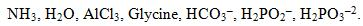Solution:
*Answer can only contain numeric values
QUESTION: 47

30 cc of M/3 HCl, 20cc of M/2 HNO3 and 40 cc of M/4 NaOH are mixed and volume was made   upto 1 dm3 . pH of resulting solution is:

Solution:
*Answer can only contain numeric values
QUESTION: 48

Maximum coordination number of Oxygen in liquid water is:

Solution:
*Answer can only contain numeric values
QUESTION: 49

As-Cl bond distance in AsCl3 is 2.20 angstrom. If radius of Chlorine atom is 0.99 Å, Then, radius (in Å) of As atom is:

Solution:
*Answer can only contain numeric values
QUESTION: 50

No of correct matches are: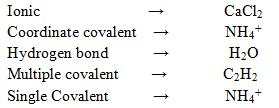Solution:
*Answer can only contain numeric values
QUESTION: 51

50.0 mL of 0.3 M HCl is mixed with 50 mL of 0.4 M NH3 solution. If pKa of NH4+ is 9.26, pH of the mixture is:

Solution:
*Answer can only contain numeric values
QUESTION: 52

if the freezing point of 0.1 molal HA(aq) is –0.2046°C, then pH of the solution is [Kf(H2O) = 1.86°C mol–1 kg]:

Solution:
*Answer can only contain numeric values
QUESTION: 53

A solution containing 30g of non-volatile solute in exactly 90g of water has a vapor pressure of    21.85 mm of Hg at 25oC. Further 18g of water is then added to the solution. The resulting       solution has then vapor pressure of 22.15 mmHg at same temp. M.wt. of solute will be:

Solution:
*Answer can only contain numeric values
QUESTION: 54

[OH] in a solution prepared by mixing equal volumes of 0.3 M methyl amine (CH3NH2, Kb = 3.7 × 10–4) and 0.60 M CH3NH3+Clis (in the multiple of 10–4 M):

Solution:
*Answer can only contain numeric values
QUESTION: 55

The Si—O—Si bond angle in Ph3SiOSiPh3 is:

Solution:
*Answer can only contain numeric values
QUESTION: 56

Number of lone pair(s) in XeOF4 is/are:

Solution:
*Answer can only contain numeric values
QUESTION: 57

No of tetrahedral units in the structure of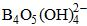are:

Solution:
*Answer can only contain numeric values
QUESTION: 58

The number (total) of σ and π bonds present in the C3O2 are:

Solution:
*Answer can only contain numeric values
QUESTION: 59

The number of isomers having non–zero dipole moment for PCl2F3 in the trigonal bipyramidal geometry is:

Solution:
*Answer can only contain numeric values
QUESTION: 60

What is the bond order in NO molecule:

Solution: